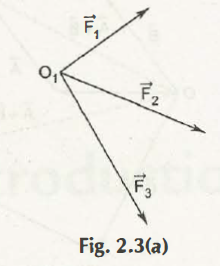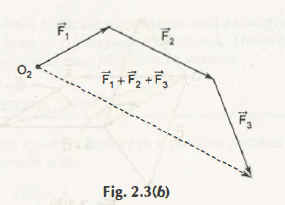Supposedly, there are two vectors A and B which are added through arrows which help to represent vectors in way where initial point of B is found on terminal point of A. It is the result of C=A + B where vector from initial point of A to terminal point of B.There are many vectors that can be added together in a manner by joining two different successive vectors from its head to tail as it is represented on the left diagram.In case the polygon is closed, then the resultant would help to obtain vector that comes with zero magnitude and there would be no direction. So, it is said to be a NULL vector or 0.

Links of Next Mechanical Engineering Topics:-### Customer Reviews

My Homework Help
Rated 5.0 out of 5 based on 510 customer reviews at